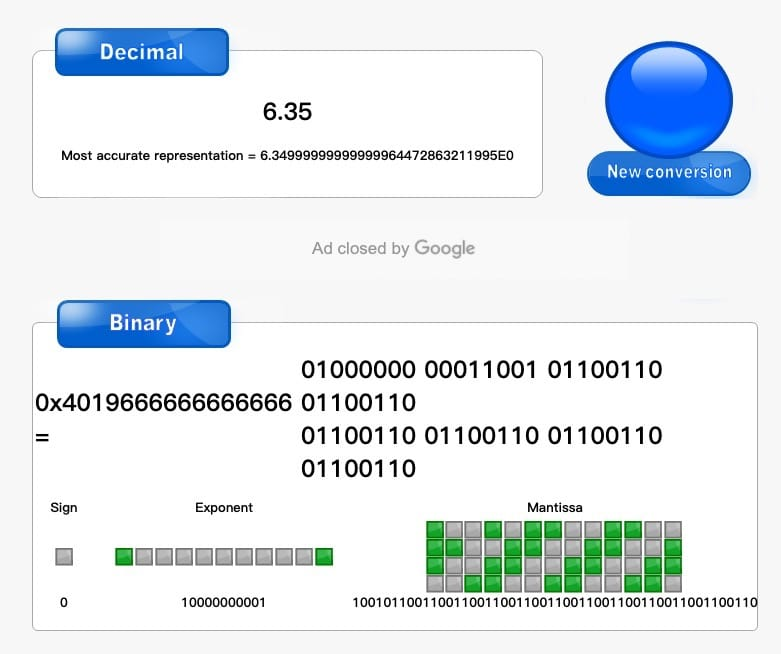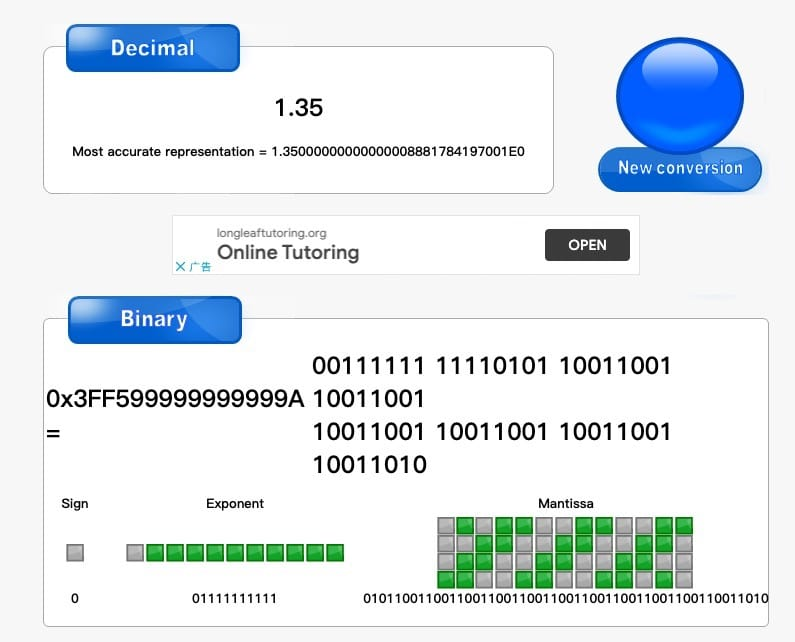### JavaScript 中的浮点数

#### 浮点数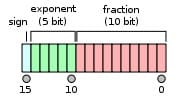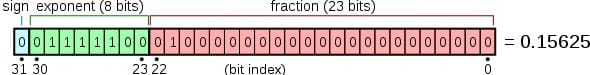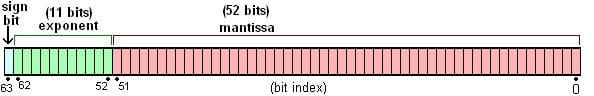​ $$V=(-1)^S×2^E×M$$

• 符号位（sign）：长度为 1 bit，其中 0 表示正数，1 表示负数

• 指数位（exponent）：表示 2 的次方数

指数为无符号整数（unsigned int），单精度类型的取值范围为 [0, 255]，双精度类型的取值范围为 [0, 2047]。在科学计数法中，指数位是允许为负数的，因而标准规定，指数位的真实值必须减去一个指数偏移量（Exponent bias），单精度类型的偏移量为 127，双精度类型的偏移量为 1023。如：

​ $$2^{10} = 2^{1033 - 1023}$$

• 指数位全为 0 时，指数位为 1-1271-1023，表示正负 0 或者无限接近于 0 的小数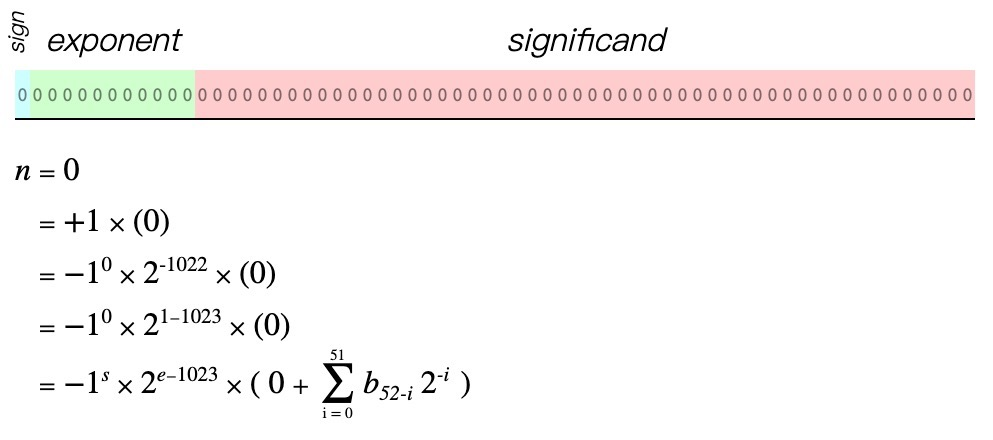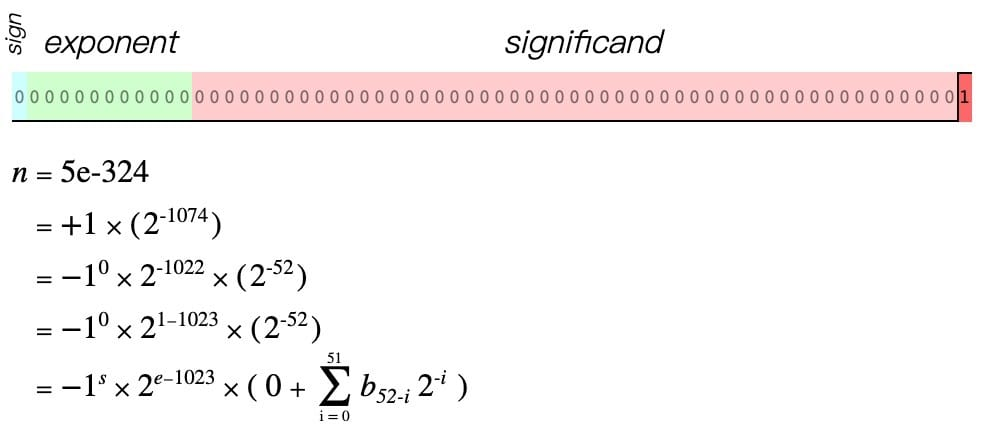• 指数全为 1 时，如果尾数位全为 1，则表示正负无穷大；尾数位不全为 0 时，表示 NaN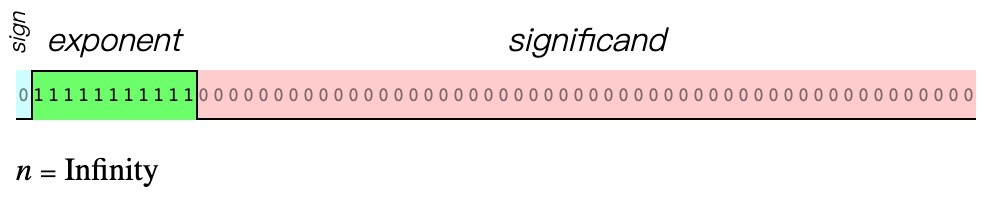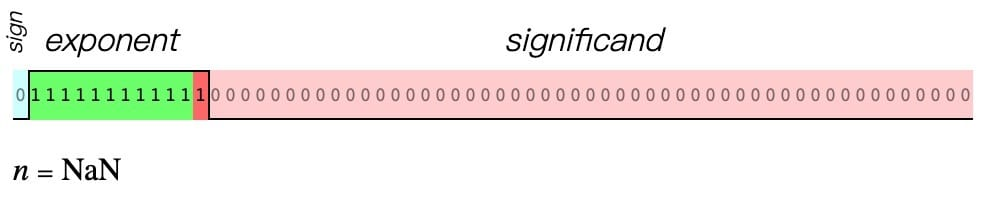• 指数不全为 0 或 1 时，采用上面的规则表示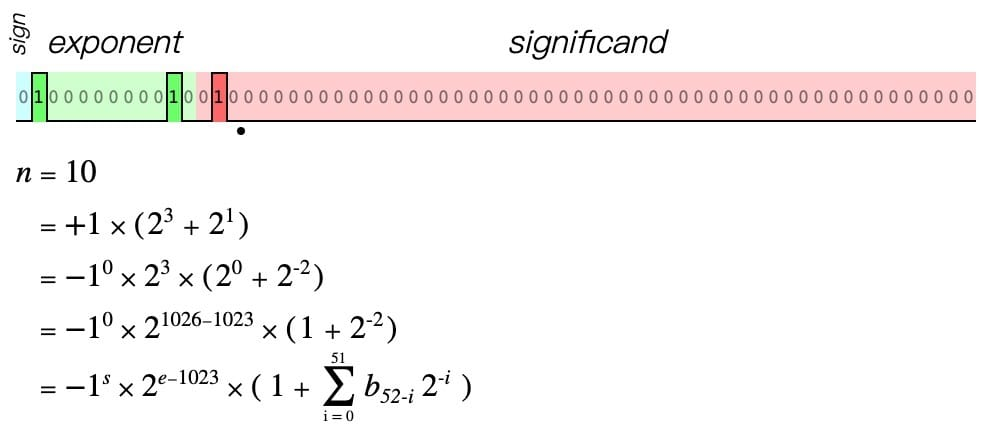• 尾数位（mantissa）：范围为 [1, 2)，即 1.xxxxxxxxx 为小数部分

IEEE 754 规定存储尾数时，默认尾数的第一位总是 1，因此可以省略 1，只保存后面的小数部分；如：1.23，只保存 23，获取的时候再把整数位 1 加上。这种存储方式可以节约 1 位长度。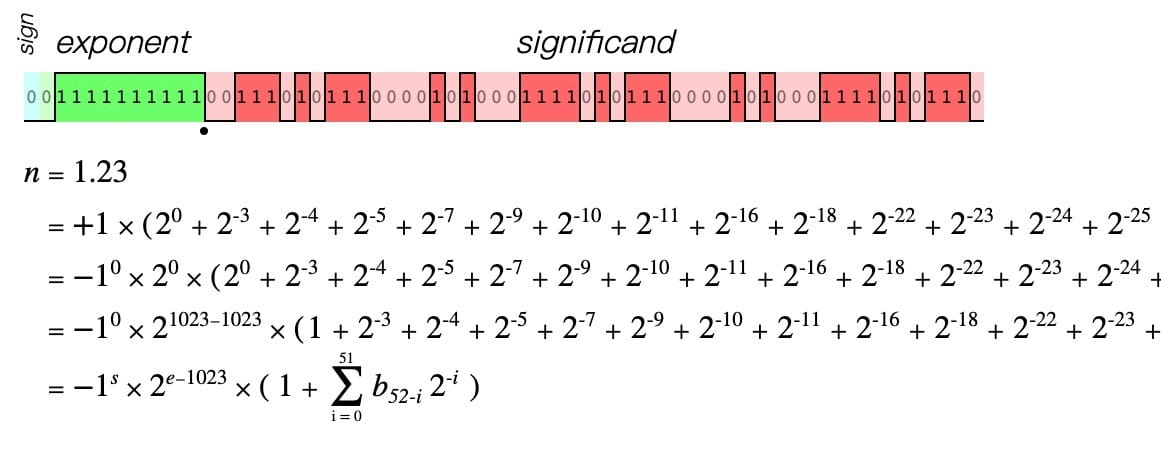#### Number 类型

##### 安全整数

​ $$Number.MAX_SAFE_INTEGER = 2^{53} - 1 = 9007199254740991$$

​ $$Number.MIN_SAFE_INTEGER = -2^{53} + 1 = -9007199254740991$$

Math.pow(2, 53)90071992547409921{53 个 0}$个1.{53个0}*2^{53}$exponent: 52 + 1023
mantissa: 000…0000 （53 个 0）

Math.pow(2, 53) + 190071992547409921{52 个 0}1$个1.{52个0}1*2^{53}$exponent: 52 + 1023
mantissa: 000…0001 （52 个 0 + 1）

Math.pow(2, 53) + 290071992547409941{51 个 0}10$个1.{51个0}10*2^{53}$exponent: 52 + 1023
mantissa: 000…0010 （51 个 0 + 10）

Math.pow(2, 53) + 390071992547409961{51 个 0}11$个1.{51个0}11*2^{53}$exponent: 52 + 1023
mantissa: 000…0011 （51 个 0 + 11）
mantissa的第 52 位和第 53 位都为 1 时进了 1 位，实际 mantissa: 50 个 0 + 10
Math.pow(2, 53) + 490071992547409961{50 个 0}100$个1.{50个0}100*2^{53}$exponent: 52 + 1023
mantissa: 000…0100 （50 个 0 + 100）

Math.pow(2, 53) + 590071992547409961{50 个 0}101$个1.{50个0}101*2^{53}$exponent: 52 + 1023，mantissa: 000…0101 （50 个 0 + 101）最后一个 1 被舍去
Math.pow(2, 53) + 690071992547409981{50 个 0}110$个1.{50个0}110*2^{53}$exponent: 52 + 1023，mantissa: 000…0110 （50 个 0 + 110）最后一个 0 被舍去
Math.pow(2, 53) + 790071992547410001{50 个 0}111$个1.{50个0}111*2^{53}$exponent: 52 + 1023，mantissa: 000…0111 （50 个 0 + 111）mantissa的第 52 位和第 53 位都为 1 时进了 1 位，实际 mantissa: 49 个 0 + 100
Math.pow(2, 53) + 890071992547410001{49 个 0}1000$个1.{49个0}1000*2^{53}$exponent: 52 + 1023，mantissa: 000…1000 （49 个 0 + 1000）最后一个 0 被舍去
Math.pow(2, 53) + 990071992547410001{49 个 0}1001$个1.{49个0}1001*2^{53}$exponent: 52 + 1023，mantissa: 000…1001 （49 个 0 + 1001）最后一个 1 被舍去
Math.pow(2, 53) + 1090071992547410021{49 个 0}1010$个1.{49个0}1010*2^{53}$exponent: 52 + 1023，mantissa: 000…1010 （49 个 0 + 1010）最后一个 0 被舍去

##### Number.EPSILON

​ $$Math.pow(2, -52) = 2.220446049250313e-16$$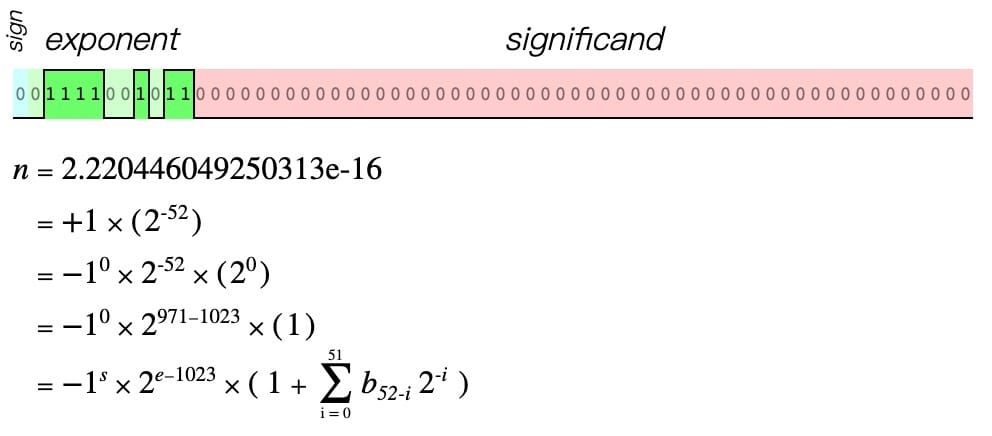ES6 在 Number 中新增了一个常量 Number.EPSILON，即 $2^{-52}$。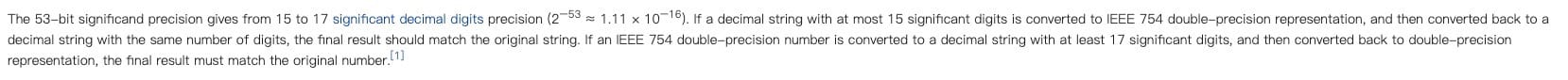#### BigInt 类型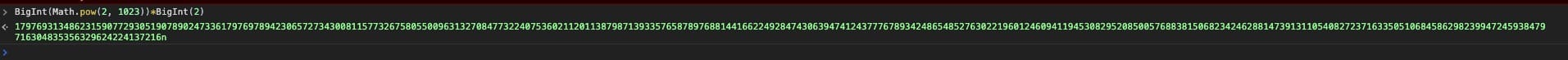#### 怪异问题

##### 0.1 + 0.2

JavaScript 中有个奇怪的现象 $0.1 + 0.2 = 0.30000000000000004$，我们来解释下具体原因：

JavaScript 中所有关于数字的运算都会先转换为二进制，再转成二进制的科学计数法，按照双精度浮点格式进行存储，然后取出取出的值并转换为二进制，最后再进行运算。

• 0.1 转换为二进制

0.1 转换为二进制后是个无限循环的数

由于尾数位只能存储 53 位，超出部分会舍弃，但 53 位数字为 1，因此会进位，最终存储为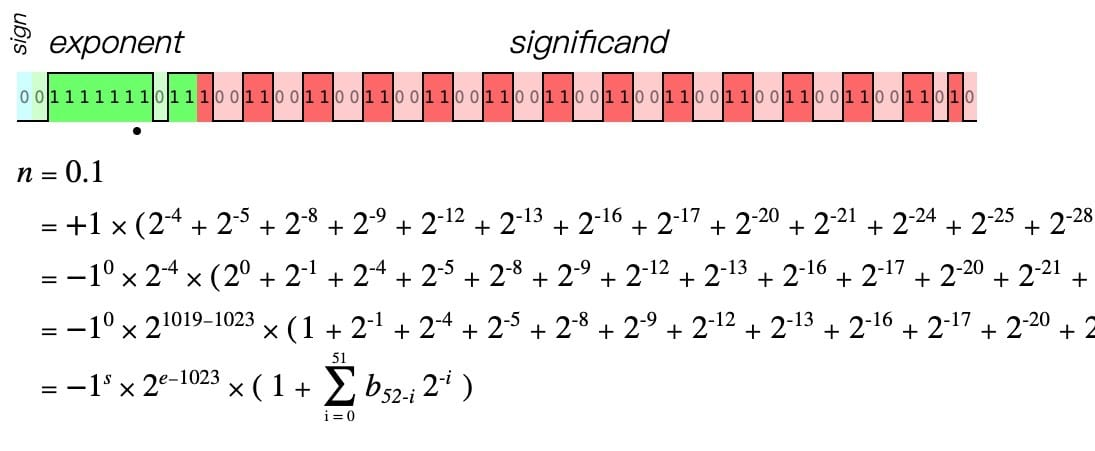• 0.2 转换为二进制

0.2 转换为二进制后也是个无限循环的数，超出部分同样舍弃

由于尾数位只能存储 53 位，超出部分会舍弃，但 53 位数字为 1，因此会进位，最终存储为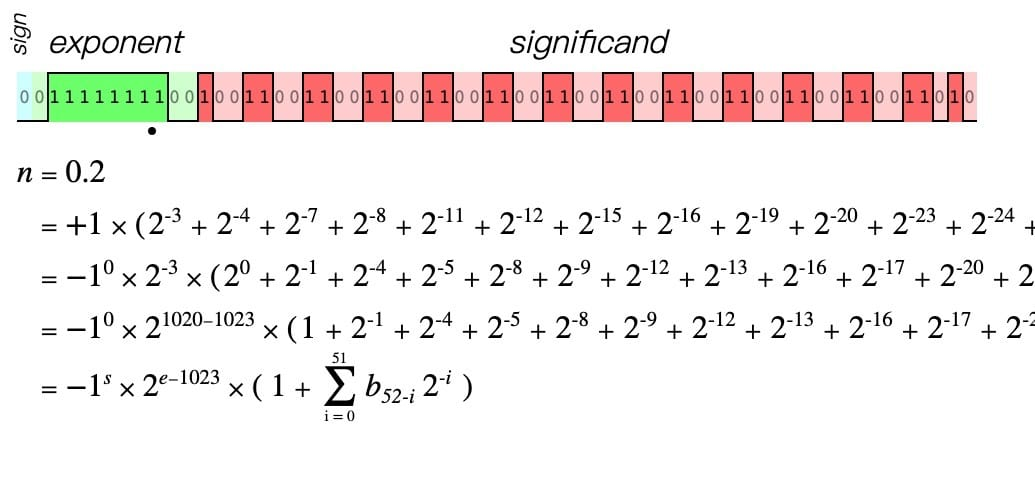• 二进制相加

从存储中取出两个数进行相加

由于在存储过程中存在舍弃和进位的原因，导致精度缺失，出现了浮点数误差。

##### 144.7 - 94.9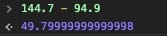• 144.7 转换为二进制存储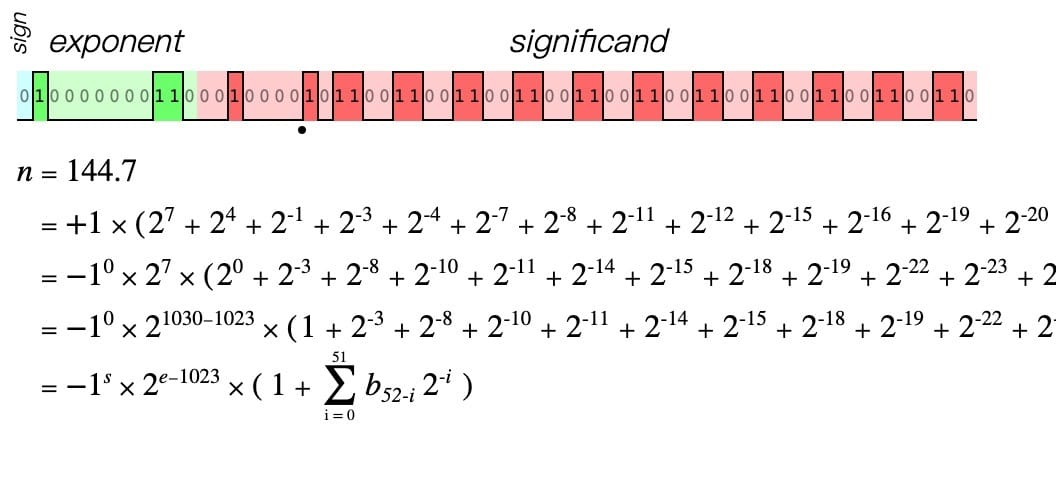• 94.9 转换为二进制存储

• 二进制相减

##### toFiexed()